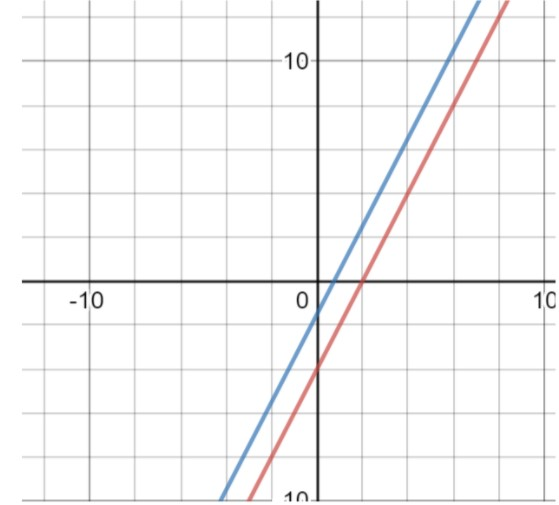QuestionAnswers

# Fill in the blanks: _____________ system of linear equation has no solutions:A. InconsistentB. ConsistentC. DependentD. Empty

Hint: A system of linear equations is either consistent or inconsistent. Their consistency depends on the ratio of their respective coefficients and the nature of the graphs represented by them. While a consistent system of linear equations have unique or infinite solutions. The inconsistent systems of linear equations have no solutions at all.

Complete step by step solution:
So, in this problem we need to find out the kind of linear equations that have no solution.
Let there be a system of two linear equations given as:
${a_1}x + {b_1}y + {c_1} = 0 \\ {a_2}x + {b_2}y + {c_2} = 0 \\$
such that the lines represents by the equations ${a_1}x + {b_1}y + {c_1} = 0$ and ${a_2}x + {b_2}y + {c_2} = 0$ respectively are parallel lines which never intersect :For a system of linear equations to have a unique solution, the lines should intersect. But since the lines in this case are parallel, it means they will never intersect anywhere and hence it will have no solution. Thus, such a system of linear equations is called inconsistent.
So we can say that an inconsistent pair of linear equations has no solution such that:
For a system of equations if $\dfrac{{{a_1}}}{{{a_2}}} = \dfrac{{{b_1}}}{{{b_2}}} \ne \dfrac{{{c_1}}}{{{c_2}}}$ then, the system of linear equations is inconsistent having no solution.
Hence an inconsistent system of linear equations has no solution.
So, our correct answer is option A.

Note: For a given pair of linear equations represented as :
${a_1}x + {b_1}y + {c_1} = 0 \\ {a_2}x + {b_2}y + {c_2} = 0 \\$
Case 1) If, $\dfrac{{{a_1}}}{{{a_2}}} \ne \dfrac{{{b_1}}}{{{b_2}}}$ , then the system of equations is consistent having a unique solution, since the lines represented by their graphs intersect at one point.
Case 2) If $\dfrac{{{a_1}}}{{{a_2}}} = \dfrac{{{b_1}}}{{{b_2}}} = \dfrac{{{c_1}}}{{{c_2}}}$, then the system of equations is consistent having infinitely many solutions, since the lines represented by their graphs are coincident.